Next: Experimental results Up: Cycling attack against faulty Previous: Fast exponentiation algorithms

## Our attack

Let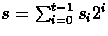be the secret key to be discovered. We suppose that exponentiations are achieved by the right-to-left square-and-multiply algorithm (Fig. 3). The attack supposes that the register containing the value of y has some permanently damaged known bits, always 0' or 1'. Let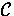and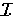denote the subsets of correct and incorrect (i.e. damaged) bits of register y , respectively. So, any value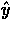stored in register y can be written as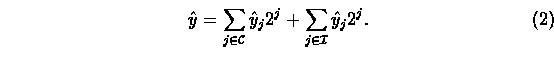From Eq. (1), the faulty signature corresponding to message m is given byLet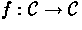be the function that maps the correct bits of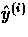to the correct bits of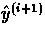. Sinceis finite, the sequence must eventually cycle. The length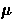of the tail and the length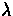of the cycle can efficiently be computed by the Floyd's algorithm [9, exercise 6 on p. 7]. This algorithm is also known as the kangaroos' method . It has the advantage of minimizing the storage requirements.

Two kangaroos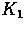and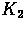cover the sequence generated by f . Kangarooprogresses in bounds of 1 unit and kangarooin bounds of 2 units. Since the sequence cycles, the two kangaroos will meet. If they meet after k bounds, then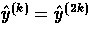. Once k has been found, it suffices to generateand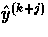for.Then,is the smallest integer j such that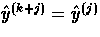.

Proof.Since, it follows that (2k-k) is a multiple of. Moreover since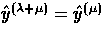,is the smallest integer j such that.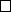lengthof the cycle is the smallest integer j such that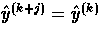. Putting all together, we obtain the Floyd's algorithm (see Fig. 5).

Figure 5: Kangaroos' method.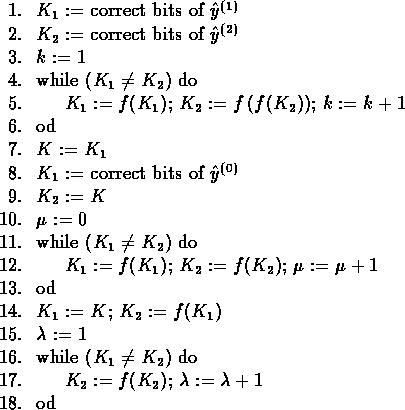To recover the bits of the secret exponent s , the pirate Carol proceeds as follows. She first chooses a random message m . Then, by the kangaroos' method, she computes the tail's lengthand the cycle's lengthof the sequence generated by the. If the sequence does not cycle, she chooses another message m and reiterates the process. The first bit of s is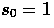since s must be odd. To find the next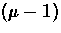bits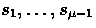, Carol asks to Alice to sign someand successively evaluatesIf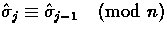then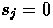;otherwise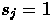.

Proof.Assume that Carol already computed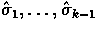and therefore knows. Then, she computes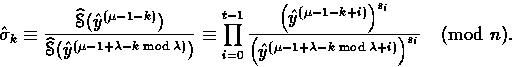Suppose that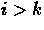. Then letting i=k+1+j with, we have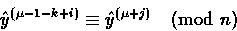andSo,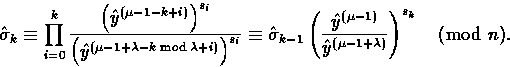There is no general recipe to find the remaining bits of s . If the lengthof the tail is short, Carol may choose another random message m to find thefirst bits of s . The next bits have to be found by exhaustive search. However, this search can be speeded up by noting that the unknown bits have to fulfill some algebraic relations. For example, the bits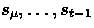must satisfy the following relations: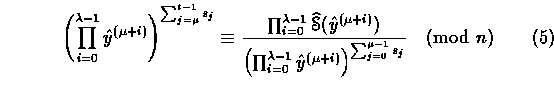andProof.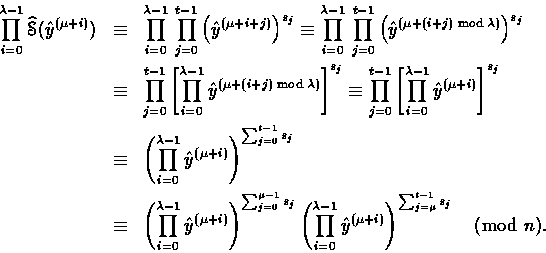Note that if, then Eq. (6) simplifies to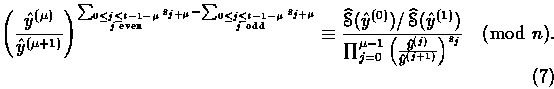Similar relations can be exhibited ifis a power of 2.Next: Experimental results Up: Cycling attack against faulty Previous: Fast exponentiation algorithms